Intermediate Algebra

# 3.3Find the Equation of a Line

Intermediate Algebra3.3 Find the Equation of a Line

### Learning Objectives

By the end of this section, you will be able to:
• Find an equation of the line given the slope and $y-intercepty-intercept$
• Find an equation of the line given the slope and a point
• Find an equation of the line given two points
• Find an equation of a line parallel to a given line
• Find an equation of a line perpendicular to a given line

### Be Prepared 3.3

Before you get started, take this readiness quiz.

1. Solve: $25(x+15).25(x+15).$
If you missed this problem, review Example 1.50.
2. Simplify: $−3(x−(−2)).−3(x−(−2)).$
If you missed this problem, review Example 1.53.
3. Solve for y: $y−3=−2(x+1).y−3=−2(x+1).$
If you missed this problem, review Example 2.31.

How do online companies know that “you may also like” a particular item based on something you just ordered? How can economists know how a rise in the minimum wage will affect the unemployment rate? How do medical researchers create drugs to target cancer cells? How can traffic engineers predict the effect on your commuting time of an increase or decrease in gas prices? It’s all mathematics.

The physical sciences, social sciences, and the business world are full of situations that can be modeled with linear equations relating two variables. To create a mathematical model of a linear relation between two variables, we must be able to find the equation of the line. In this section, we will look at several ways to write the equation of a line. The specific method we use will be determined by what information we are given.

### Find an Equation of the Line Given the Slope and y-Intercept

We can easily determine the slope and intercept of a line if the equation is written in slope-intercept form, $y=mx+b.y=mx+b.$ Now we will do the reverse—we will start with the slope and y-intercept and use them to find the equation of the line.

### Example 3.24

Find the equation of a line with slope $−9−9$ and y-intercept $(0,−4).(0,−4).$

### Try It 3.47

Find the equation of a line with slope $2525$ and y-intercept $(0,4).(0,4).$

### Try It 3.48

Find the equation of a line with slope $−1−1$ and y-intercept $(0,−3).(0,−3).$

Sometimes, the slope and intercept need to be determined from the graph.

### Example 3.25

Find the equation of the line shown in the graph.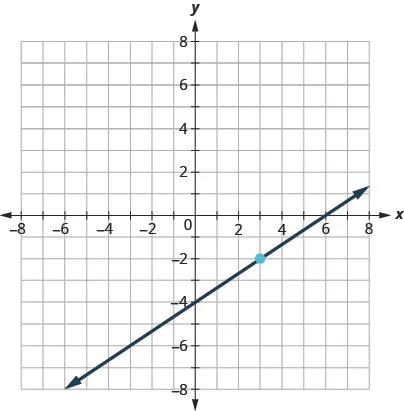### Try It 3.49

Find the equation of the line shown in the graph.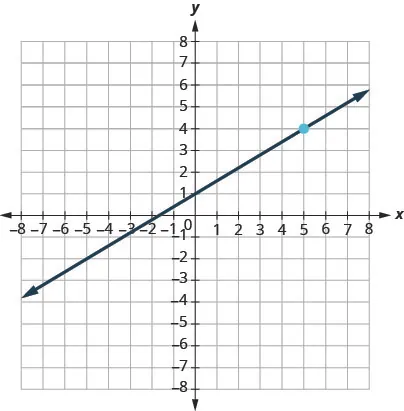### Try It 3.50

Find the equation of the line shown in the graph.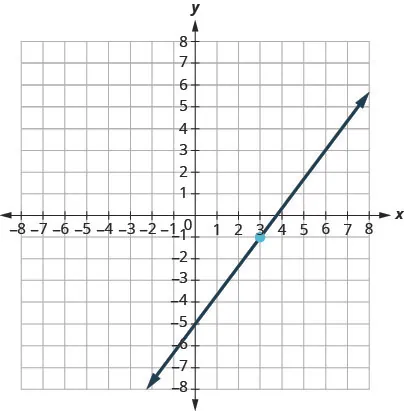### Find an Equation of the Line Given the Slope and a Point

Finding an equation of a line using the slope-intercept form of the equation works well when you are given the slope and y-intercept or when you read them off a graph. But what happens when you have another point instead of the y-intercept?

We are going to use the slope formula to derive another form of an equation of the line.

Suppose we have a line that has slope m and that contains some specific point $(x1,y1)(x1,y1)$ and some other point, which we will just call $(x,y).(x,y).$ We can write the slope of this line and then change it to a different form.

$m=y−y1x−x1 Multiply both sides of the equation byx−x1.m(x−x1)=(y−y1x−x1)(x−x1) Simplify.m(x−x1)=y−y1 Rewrite the equation with theyterms on the left.y−y1=m(x−x1)m=y−y1x−x1 Multiply both sides of the equation byx−x1.m(x−x1)=(y−y1x−x1)(x−x1) Simplify.m(x−x1)=y−y1 Rewrite the equation with theyterms on the left.y−y1=m(x−x1)$

This format is called the point-slope form of an equation of a line.

### Point-slope Form of an Equation of a Line

The point-slope form of an equation of a line with slope m and containing the point $(x1,y1)(x1,y1)$ is:

$y−y1=m(x−x1)y−y1=m(x−x1)$

We can use the point-slope form of an equation to find an equation of a line when we know the slope and at least one point. Then, we will rewrite the equation in slope-intercept form. Most applications of linear equations use the the slope-intercept form.

### Example 3.26

#### How to Find an Equation of a Line Given a Point and the Slope

Find an equation of a line with slope $m=−13m=−13$ that contains the point $(6,−4).(6,−4).$ Write the equation in slope-intercept form.

### Try It 3.51

Find the equation of a line with slope $m=−25m=−25$ and containing the point $(10,−5).(10,−5).$

### Try It 3.52

Find the equation of a line with slope $m=−34,m=−34,$ and containing the point $(4,−7).(4,−7).$

We list the steps for easy reference.

### How To

#### To find an equation of a line given the slope and a point.

1. Step 1. Identify the slope.
2. Step 2. Identify the point.
3. Step 3. Substitute the values into the point-slope form, $y−y1=m(x−x1).y−y1=m(x−x1).$
4. Step 4. Write the equation in slope-intercept form.

### Example 3.27

Find an equation of a horizontal line that contains the point $(−2,−6).(−2,−6).$ Write the equation in slope-intercept form.

### Try It 3.53

Find the equation of a horizontal line containing the point $(−3,8).(−3,8).$

### Try It 3.54

Find the equation of a horizontal line containing the point $(−1,4).(−1,4).$

### Find an Equation of the Line Given Two Points

When real-world data is collected, a linear model can be created from two data points. In the next example we’ll see how to find an equation of a line when just two points are given.

So far, we have two options for finding an equation of a line: slope-intercept or point-slope. When we start with two points, it makes more sense to use the point-slope form.

But then we need the slope. Can we find the slope with just two points? Yes. Then, once we have the slope, we can use it and one of the given points to find the equation.

### Example 3.28

#### How to Find the Equation of a Line Given Two Points

Find an equation of a line that contains the points $(−3,−1)(−3,−1)$ and $(2,−2)(2,−2)$ Write the equation in slope-intercept form.

### Try It 3.55

Find the equation of a line containing the points $(−2,−4)(−2,−4)$ and $(1,−3).(1,−3).$

### Try It 3.56

Find the equation of a line containing the points $(−4,−3)(−4,−3)$ and $(1,−5).(1,−5).$

The steps are summarized here.

### How To

#### To find an equation of a line given two points.

1. Step 1. Find the slope using the given points. $m=y2−y1x2−x1m=y2−y1x2−x1$
2. Step 2. Choose one point.
3. Step 3. Substitute the values into the point-slope form: $y−y1=m(x−x1).y−y1=m(x−x1).$
4. Step 4. Write the equation in slope-intercept form.

### Example 3.29

Find an equation of a line that contains the points $(−3,5)(−3,5)$ and $(−3,4).(−3,4).$ Write the equation in slope-intercept form.

### Try It 3.57

Find the equation of a line containing the points $(5,1)(5,1)$ and $(5,−4).(5,−4).$

### Try It 3.58

Find the equaion of a line containing the points $(−4,4)(−4,4)$ and $(−4,3).(−4,3).$

We have seen that we can use either the slope-intercept form or the point-slope form to find an equation of a line. Which form we use will depend on the information we are given.

To Write an Equation of a Line
If given: Use: Form:
Slope and y-intercept slope-intercept $y=mx+by=mx+b$
Slope and a point point-slope $y−y1=m(x−x1)y−y1=m(x−x1)$
Two points point-slope $y−y1=m(x−x1)y−y1=m(x−x1)$

### Find an Equation of a Line Parallel to a Given Line

Suppose we need to find an equation of a line that passes through a specific point and is parallel to a given line. We can use the fact that parallel lines have the same slope. So we will have a point and the slope—just what we need to use the point-slope equation.

First, let’s look at this graphically.

This graph shows $y=2x−3.y=2x−3.$ We want to graph a line parallel to this line and passing through the point $(−2,1).(−2,1).$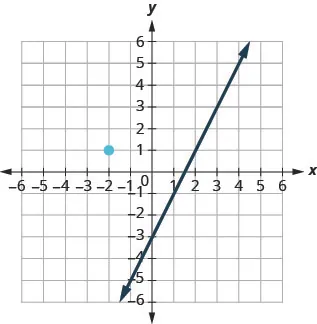We know that parallel lines have the same slope. So the second line will have the same slope as $y=2x−3.y=2x−3.$ That slope is $m∥=2.m∥=2.$ We’ll use the notation $m∥m∥$ to represent the slope of a line parallel to a line with slope m. (Notice that the subscript || looks like two parallel lines.)

The second line will pass through $(−2,1)(−2,1)$ and have $m=2.m=2.$

To graph the line, we start at$(−2,1)(−2,1)$ and count out the rise and run.

With $m=2m=2$ (or $m=21m=21$), we count out the rise 2 and the run 1. We draw the line, as shown in the graph.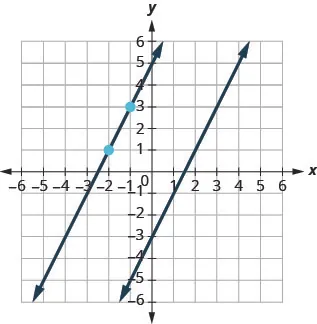Do the lines appear parallel? Does the second line pass through$(−2,1)?(−2,1)?$

We were asked to graph the line, now let’s see how to do this algebraically.

We can use either the slope-intercept form or the point-slope form to find an equation of a line. Here we know one point and can find the slope. So we will use the point-slope form.

### Example 3.30

#### How to Find the Equation of a Line Parallel to a Given Line and a Point

Find an equation of a line parallel to $y=2x−3y=2x−3$ that contains the point $(−2,1).(−2,1).$ Write the equation in slope-intercept form.

### Try It 3.59

Find an equation of a line parallel to the line $y=3x+1y=3x+1$ that contains the point $(4,2).(4,2).$ Write the equation in slope-intercept form.

### Try It 3.60

Find an equation of a line parallel to the line $y=12x−3y=12x−3$ that contains the point $(6,4).(6,4).$

Write the equation in slope-intercept form.

### How To

#### Find an equation of a line parallel to a given line.

1. Step 1. Find the slope of the given line.
2. Step 2. Find the slope of the parallel line.
3. Step 3. Identify the point.
4. Step 4. Substitute the values into the point-slope form: $y−y1=m(x−x1).y−y1=m(x−x1).$
5. Step 5. Write the equation in slope-intercept form.

### Find an Equation of a Line Perpendicular to a Given Line

Now, let’s consider perpendicular lines. Suppose we need to find a line passing through a specific point and which is perpendicular to a given line. We can use the fact that perpendicular lines have slopes that are negative reciprocals. We will again use the point-slope equation, like we did with parallel lines.

This graph shows $y=2x−3.y=2x−3.$ Now, we want to graph a line perpendicular to this line and passing through $(−2,1).(−2,1).$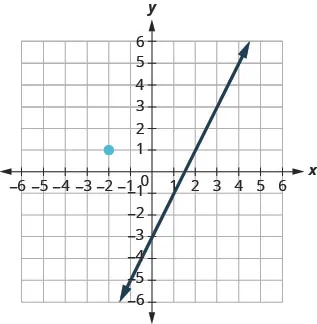We know that perpendicular lines have slopes that are negative reciprocals.

We’ll use the notation $m⊥m⊥$ to represent the slope of a line perpendicular to a line with slope m. (Notice that the subscript $⊥⊥$ looks like the right angles made by two perpendicular lines.)

$y=2x−3perpendicular line m=2m⊥=−12y=2x−3perpendicular line m=2m⊥=−12$

We now know the perpendicular line will pass through $(−2,1)(−2,1)$ with $m⊥=−12.m⊥=−12.$

To graph the line, we will start at $(−2,1)(−2,1)$ and count out the rise $−1−1$ and the run 2. Then we draw the line.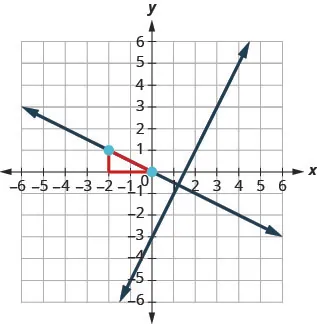Do the lines appear perpendicular? Does the second line pass through$(−2,1)?(−2,1)?$

We were asked to graph the line, now, let’s see how to do this algebraically.

We can use either the slope-intercept form or the point-slope form to find an equation of a line. In this example we know one point, and can find the slope, so we will use the point-slope form.

### Example 3.31

#### How to Find the Equation of a Line Perpendicular to a Given Line and a Point

Find an equation of a line perpendicular to $y=2x−3y=2x−3$ that contains the point $(−2,1).(−2,1).$ Write the equation in slope-intercept form.

### Try It 3.61

Find an equation of a line perpendicular to the line $y=3x+1y=3x+1$ that contains the point $(4,2).(4,2).$ Write the equation in slope-intercept form.

### Try It 3.62

Find an equation of a line perpendicular to the line $y=12x−3y=12x−3$ that contains the point $(6,4).(6,4).$ Write the equation in slope-intercept form.

### How To

#### Find an equation of a line perpendicular to a given line.

1. Step 1. Find the slope of the given line.
2. Step 2. Find the slope of the perpendicular line.
3. Step 3. Identify the point.
4. Step 4. Substitute the values into the point-slope form, $y−y1=m(x−x1).y−y1=m(x−x1).$
5. Step 5. Write the equation in slope-intercept form.

### Example 3.32

Find an equation of a line perpendicular to $x=5x=5$ that contains the point $(3,−2).(3,−2).$ Write the equation in slope-intercept form.

### Try It 3.63

Find an equation of a line that is perpendicular to the line $x=4x=4$ that contains the point $(4,−5).(4,−5).$. Write the equation in slope-intercept form.

### Try It 3.64

Find an equation of a line that is perpendicular to the line $x=2x=2$ that contains the point $(2,−1).(2,−1).$ Write the equation in slope-intercept form.

In Example 3.32, we used the point-slope form to find the equation. We could have looked at this in a different way.

We want to find a line that is perpendicular to $x=5x=5$ that contains the point $(3,−2).(3,−2).$ This graph shows us the line$x=5x=5$ and the point $(3,−2).(3,−2).$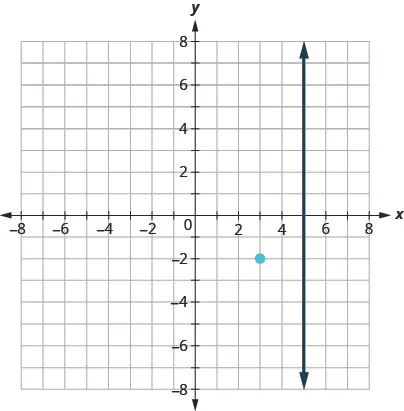We know every line perpendicular to a vertical line is horizontal, so we will sketch the horizontal line through $(3,−2).(3,−2).$Do the lines appear perpendicular?

If we look at a few points on this horizontal line, we notice they all have y-coordinates of $−2.−2.$ So, the equation of the line perpendicular to the vertical line $x=5x=5$ is $y=−2.y=−2.$

### Example 3.33

Find an equation of a line that is perpendicular to $y=−3y=−3$ that contains the point $(−3,5).(−3,5).$ Write the equation in slope-intercept form.

### Try It 3.65

Find an equation of a line that is perpendicular to the line $y=1y=1$ that contains the point $(−5,1).(−5,1).$ Write the equation in slope-intercept form.

### Try It 3.66

Find an equation of a line that is perpendicular to the line $y=−5y=−5$ that contains the point $(−4,−5).(−4,−5).$ Write the equation in slope-intercept form.

### Media

Access these online resources for additional instruction and practice with finding the equation of a line.

### Section 3.3 Exercises

#### Practice Makes Perfect

Find an Equation of the Line Given the Slope and y-Intercept

In the following exercises, find the equation of a line with given slope and y-intercept. Write the equation in slope-intercept form.

155.

slope 3 and
$yy$-intercept $(0,5)(0,5)$

156.

slope 8 and
y-intercept $(0,−6)(0,−6)$

157.

slope $−3−3$ and
$yy$-intercept $(0,−1)(0,−1)$

158.

slope $−1−1$ and
$yy$-intercept $(0,3)(0,3)$

159.

slope $1515$ and
$yy$-intercept $(0,−5)(0,−5)$

160.

slope $−34−34$ and
$yy$-intercept $(0,−2)(0,−2)$

161.

slope 0 and
$yy$-intercept $(0,−1)(0,−1)$

162.

slope $−4−4$ and
$yy$-intercept $(0,0)(0,0)$

In the following exercises, find the equation of the line shown in each graph. Write the equation in slope-intercept form.

163.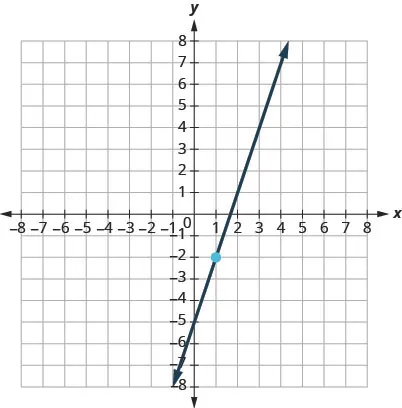164.165.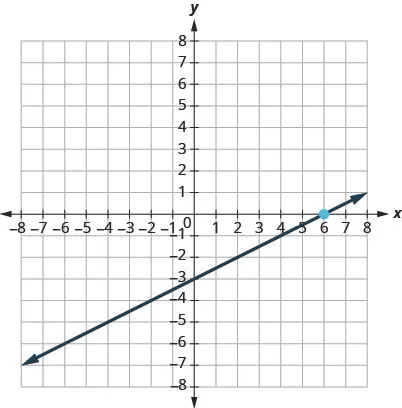166.167.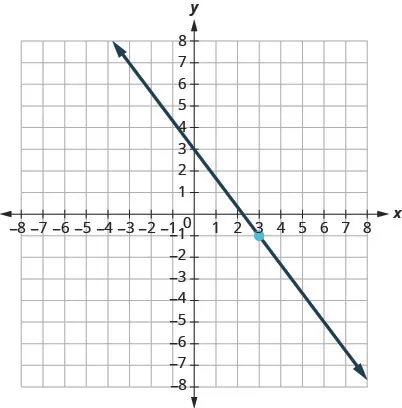168.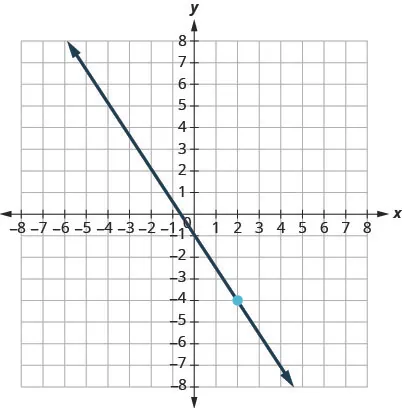169.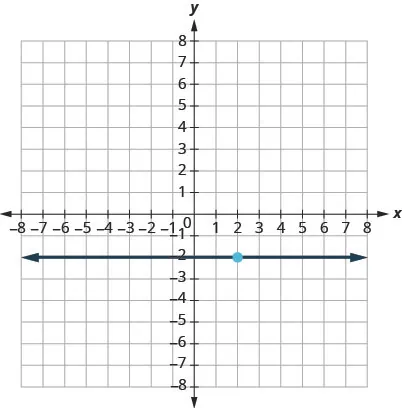170.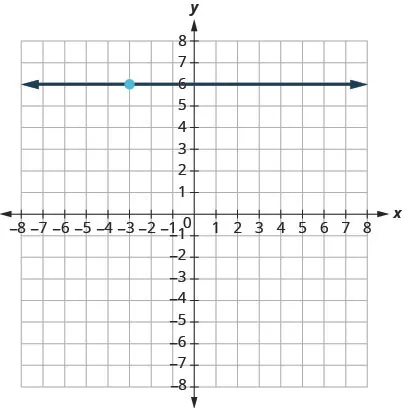Find an Equation of the Line Given the Slope and a Point

In the following exercises, find the equation of a line with given slope and containing the given point. Write the equation in slope-intercept form.

171.

$m=58,m=58,$ point $(8,3)(8,3)$

172.

$m=56,m=56,$ point $(6,7)(6,7)$

173.

$m=−35,m=−35,$ point $(10,−5)(10,−5)$

174.

$m=−34,m=−34,$ point $(8,−5)(8,−5)$

175.

$m=−32,m=−32,$ point $(−4,−3)(−4,−3)$

176.

$m=−52,m=−52,$ point $(−8,−2)(−8,−2)$

177.

$m=−7,m=−7,$ point $(−1,−3)(−1,−3)$

178.

$m=−4,m=−4,$ point $(−2,−3)(−2,−3)$

179.

Horizontal line containing $(−2,5)(−2,5)$

180.

Horizontal line containing $(−2,−3)(−2,−3)$

181.

Horizontal line containing $(−1,−7)(−1,−7)$

182.

Horizontal line containing $(4,−8)(4,−8)$

Find an Equation of the Line Given Two Points

In the following exercises, find the equation of a line containing the given points. Write the equation in slope-intercept form.

183.

$(2,6)(2,6)$ and $(5,3)(5,3)$

184.

$(4,3)(4,3)$ and $(8,1)(8,1)$

185.

$(−3,−4)(−3,−4)$ and $(5−2).(5−2).$

186.

$(−5,−3)(−5,−3)$ and $(4,−6).(4,−6).$

187.

$(−1,3)(−1,3)$ and $(−6,−7).(−6,−7).$

188.

$(−2,8)(−2,8)$ and $(−4,−6).(−4,−6).$

189.

$(0,4)(0,4)$ and $(2,−3).(2,−3).$

190.

$(0,−2)(0,−2)$ and $(−5,−3).(−5,−3).$

191.

$(7,2)(7,2)$ and $(7,−2).(7,−2).$

192.

$(−2,1)(−2,1)$ and $(−2,−4).(−2,−4).$

193.

$(3,−4)(3,−4)$ and $(5,−4).(5,−4).$

194.

$(−6,−3)(−6,−3)$ and $(−1,−3)(−1,−3)$

Find an Equation of a Line Parallel to a Given Line

In the following exercises, find an equation of a line parallel to the given line and contains the given point. Write the equation in slope-intercept form.

195.

line $y=4x+2,y=4x+2,$
point $(1,2)(1,2)$

196.

line $y=−3x−1,y=−3x−1,$
point $(2,−3).(2,−3).$

197.

line $2x−y=6,2x−y=6,$
point $(3,0).(3,0).$

198.

line $2x+3y=6,2x+3y=6,$
point $(0,5).(0,5).$

199.

line $x=−4,x=−4,$
point $(−3,−5).(−3,−5).$

200.

line $x−2=0,x−2=0,$
point $(1,−2)(1,−2)$

201.

line $y=5,y=5,$
point $(2,−2)(2,−2)$

202.

line $y+2=0,y+2=0,$
point $(3,−3)(3,−3)$

Find an Equation of a Line Perpendicular to a Given Line

In the following exercises, find an equation of a line perpendicular to the given line and contains the given point. Write the equation in slope-intercept form.

203.

line $y=−2x+3,y=−2x+3,$
point $(2,2)(2,2)$

204.

line $y=−x+5,y=−x+5,$
point $(3,3)(3,3)$

205.

line $y=34x−2,y=34x−2,$
point $(−3,4)(−3,4)$

206.

line $y=23x−4,y=23x−4,$
point $(2,−4)(2,−4)$

207.

line $2x−3y=8,2x−3y=8,$
point $(4,−1)(4,−1)$

208.

line $4x−3y=5,4x−3y=5,$
point $(−3,2)(−3,2)$

209.

line $2x+5y=6,2x+5y=6,$
point $(0,0)(0,0)$

210.

line $4x+5y=−3,4x+5y=−3,$
point $(0,0)(0,0)$

211.

line $x=3,x=3,$
point $(3,4)(3,4)$

212.

line $x=−5,x=−5,$
point $(1,−2)(1,−2)$

213.

line $x=7,x=7,$
point $(−3,−4)(−3,−4)$

214.

line $x=−1,x=−1,$
point $(−4,0)(−4,0)$

215.

line $y−3=0,y−3=0,$
point $(−2,−4)(−2,−4)$

216.

line $y−6=0,y−6=0,$
point $(−5,−3)(−5,−3)$

217.

line y-axis,
point $(3,4)(3,4)$

218.

line y-axis,
point $(2,1)(2,1)$

Mixed Practice

In the following exercises, find the equation of each line. Write the equation in slope-intercept form.

219.

Containing the points $(4,3)(4,3)$ and $(8,1)(8,1)$

220.

Containing the points $(−2,0)(−2,0)$ and $(−3,−2)(−3,−2)$

221.

$m=16,m=16,$ containing point $(6,1)(6,1)$

222.

$m=56,m=56,$ containing point $(6,7)(6,7)$

223.

Parallel to the line $4x+3y=6,4x+3y=6,$ containing point $(0,−3)(0,−3)$

224.

Parallel to the line $2x+3y=6,2x+3y=6,$ containing point $(0,5)(0,5)$

225.

$m=−34,m=−34,$ containing point $(8,−5)(8,−5)$

226.

$m=−35,m=−35,$ containing point $(10,−5)(10,−5)$

227.

Perpendicular to the line $y−1=0,y−1=0,$ point $(−2,6)(−2,6)$

228.

Perpendicular to the line y-axis, point $(−6,2)(−6,2)$

229.

Parallel to the line $x=−3,x=−3,$ containing point $(−2,−1)(−2,−1)$

230.

Parallel to the line $x=−4,x=−4,$ containing point $(−3,−5)(−3,−5)$

231.

Containing the points $(−3,−4)(−3,−4)$ and $(2,−5)(2,−5)$

232.

Containing the points $(−5,−3)(−5,−3)$ and $(4,−6)(4,−6)$

233.

Perpendicular to the line $x−2y=5,x−2y=5,$ point $(−2,2)(−2,2)$

234.

Perpendicular to the line $4x+3y=1,4x+3y=1,$ point $(0,0)(0,0)$

#### Writing Exercises

235.

Why are all horizontal lines parallel?

236.

Explain in your own words why the slopes of two perpendicular lines must have opposite signs.

#### Self Check

After completing the exercises, use this checklist to evaluate your mastery of the objectives of this section.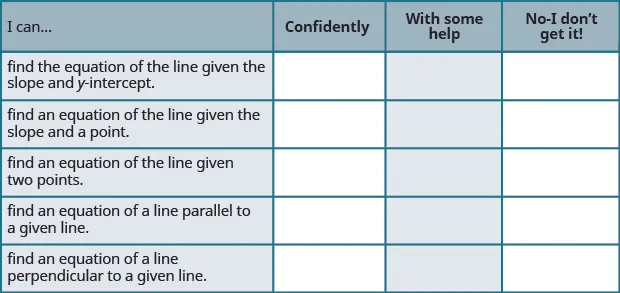What does this checklist tell you about your mastery of this section? What steps will you take to improve?

Order a print copy

As an Amazon Associate we earn from qualifying purchases.